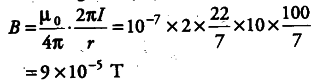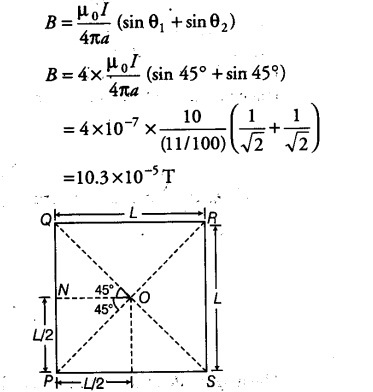# Two wires A and B have the same length equal to 44 cm

Two wires A and B have the same length equal to 44 cm and carry a current of 10 A each.
Wire A is bent into a circle and wire Bis bent into a square. .
(i) Obtain the magnitudes of the fields at the centres of the two wires.
(ii) Which wire produces a greater magnetic field at its centre?

Given, / = 10 A, length of each wire = 44 cm
(i) Let r be the radius of wire A when it is bent into a circle.
2$\pi$r = 44
r = 7/100 m
Magnetic field at the centre of the circular coil carrying current is given byWhen another wire is. bent into a square of each side L, then
4L = 44
L = 11 cm = 0.11 m
Since, -magnetic field - induction at a point, at perpendicular distance a from the linear conductor carrying current is given by(ii) The magnedc field due to a square will be more than .that due to a circle of same perimeter.